Search IntMath
Close

450+ Math Lessons written by Math Professors and Teachers

5 Million+ Students Helped Each Year

1200+ Articles Written by Math Educators and Enthusiasts

Simplifying and Teaching Math for Over 23 Years

# Roller Door problem

By Murray Bourne, 28 Nov 2012

In an earlier article, I discussed the Length of an Archimedean Spiral. We saw we can find the length of such a spiral using integration, once we know the equation of the spiral and the beginning and end points. The equation for an Archimedean Spiral is in polar form, and is determined by an angle θ, the amount of turn of the spiral.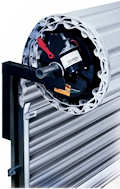Roller door.
[Image source]

Brent, an engineer with a roller door company, asked an interesting question in the comments for that article. He posed a "real-life" issue he faced.

His problem is the reverse. He knows length of the spiral already (it's the height of the roller door, plus the bit that's wrapped around the drum wheel), but he needs to know the number of turns of the drum for the given height of the door and radius of the drum.

This is not such a straightforward problem.

I suggested he could produce a table for already known door heights and number of turns. and then use interpolation to find the required number of turns (at least approximately).

Brent also turned to PhysicsForums to ask for help. He wrote:

Hi,

I'm an engineer designing a spring system for a garage roller door. I need to know the number of turns of the door for all the size combinations.

I've found this page which gives a good equation for finding the length if you know the number of turns, starting radius and gap between spirals: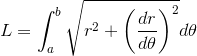The equation of the spiral is r = x + yθ, with x = starting radius, y = gap/(), and to find L we're taking the integral from a = 0 to b = 2πn (where n = turns).

When you know n, this is straightfoward, and even I could work that out. But it's been a decade since I've done anything like this, so I was wondering if anyone could help me solve for n in this integral: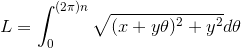My way of solving this is to find L for n=1,2,3,4,5 etc, graph it in Excel and use "find trendline" to get an equation. Any help appreciated, thanks.

The response there was not very helpful.

Brent provided his Excel spreadsheet so I could see what he was doing.

## Specific Example

Brent's roller door has a barrel radius of 127.5 cm and the thickness of the door slats (called the "curtain") is 4.0 cm. This means the radius will increase by 4.0 cm for each turn of the barrel.

So x = 127.5 and y = 4/(2π)=0.6366 in this case.

The following daigram (not to scale) indicates our situation: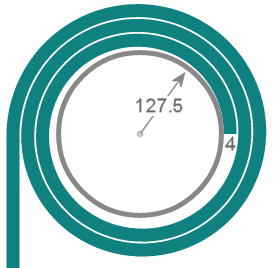Applying the integration formula above, we have the length of the curtain wrapped around the barrel for 1 turn: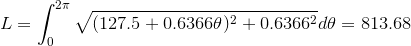Similarly, for 2 turns (that is, 0 to 4π), we have: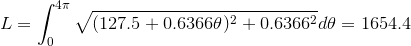Continuing on for 3 up to 6 turns, we obtain the following values:

Turns Length
0
0
1
814
2
1654
3
2516
4
3406
5
4320
6
5259

Next, we use Excel to plot these values and to draw a trendline through them. We choose "polynomial of degree 2" because a consideration of the integral and an inspection of the Excel graph (when extrapolating beyond 6 turns) shows it is parabolic.

Excel can tell us the equation for the graph produced, and it is included on the chart.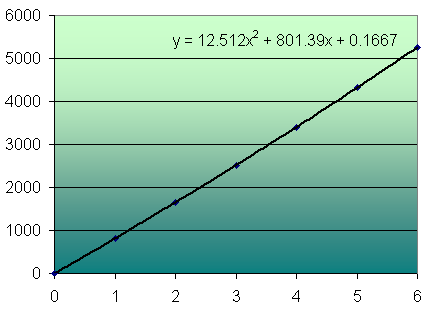We need to translate the "y" and "x" variables on Excel's chart for our own situation. The vertical scale is the length of the curtain, and the horizontal scale is the number of turns. Also, the "0.1667" value is a rounding error, which we can discard.

So in our case, we would write the Length of the curtain (L) for the number of turns, n as: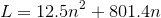Solving for n (using the quadratic formula) gives us 2 solutions. The first one is: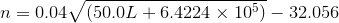The second solution gives a negative result for n, so we discard it.

We now check our work (by comparing a known value).

If the length is 3406 cm, then the number of turns given by our new formula is: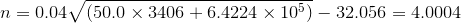This is certainly close to the 4 turns we expected.

So Brent could now use this formula to find the number of turns for any roller door's curtain length given a barrel radius of 127.5 cm and a curtain thickness of 4 cm.

## Linear Solution

The Excel graph for the range of turns 0 to 6 is very nearly linear. Using the "Linear" trendline option in Excel gives the model for the Length given the number of turns as:

L = 876.5n - 62.4

Solving for n gives: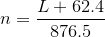Applying this simpler formula to some of our known values gives:

For length L = 814, number of turns, n = 0.99989. (expected 1)

For length L = 1654, number of turns, n = 1.9582. (expected 2)

For length L = 5529, number of turns, n = 6.0712. (expected 6)

So for lengths of the roller door curtain less than about 10 m, this simpler linear model gives acceptable results.

## Other solutions

Neil provided another solution in the comments.

He proposed: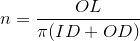where

n = number of turns

OL = overall length

ID = inner diameter of the spiral

OD = outer diameter of the spiral

However, Brent pointed out this can't be used because he doesn't know the outer diameter until he knows the number of turns.

Jacob then weighed in with another proposal, which involved taking an average of the inner and outer radius and then applying the circumference of a circle to that average.

Jacob suggests if we don't know the number of turns, we can use this formula to find it:Applying that formula to our example above, with y = 4 and ID = 127.5×2 = 255 and our known case where L = 3406 gives: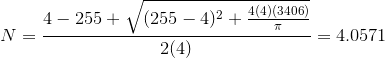This is very close to the 4 turns we expected.

Applying the formula for another known case, L = 5259, we obtain 6.08 turns, nicely close to the expected value of 6.

## Conclusion

Brent has a choice of formulas to use, all of which give acceptable results. The linear model is the easiest.

Parabolic model:Linear model:The model suggested by Neil and refined by Jacob: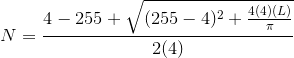Here is a table that shows the values from the given formulas.

Length
(via calculus)
Turns
(original)
Turns
(parabolic model)
Turns
(linear model)
Turns
(Neil/Jacob model)
0 0 0 0 0
814 1 1.0001 0.9999 1.0158
1654 2 2.0014 1.9582 2.0318
2516 3 2.9992 2.9417 3.0431
3406 4 4.0004 3.9571 4.0571
4320 5 5.0005 4.9999 5.069
5259 6 6.0006 6.0712 6.0802

### 9 Comments on “Roller Door problem”

1. Thomas A Buckley says:

Two different simple non calculus methods yielded identical results, which differ greatly from the table used to derive the concluding solution above. So calculus people, recheck!

For example you say starting radius x = 127.5 cm. Taking center of door thickness for length, allowing inner shortening to be balanced by outer lengthening when rolled,
effective starting radius = 127.5 + 4/2 = 129.5 cm!

.....................................Length cm....Length cm
1.........................2Pi(127.5+2)=814........880
2............2Pi(127.5+6)+814=1652.......1917
3.....2Pi(127.5+10)+1652=2516.......3111
4.....2Pi(127.5+14)+2516=3405.......4462
5.....2Pi(127.5+18)+3405=4320.......5970
6.....2Pi(127.5+22)+4320=5259.......7636
After the 1st turn each diameter increases by 4 cm.
OK, so my method is NOT exact, but it should not be so different from the results obtained by calculus.

2nd Method.
Number of Turns n
= [SqRt( Lt/pi + r^2)-r]/t

L = Door length, t = door thickness, r = barrel radius.

CSA of door = Lt
This area must fit in the space between concentric circles.
Taking R as radius when door is wound on barrel, then
Lt = piR^2 - pir^2 . . ie both areas are the same.
R = SqRt( Lt/pi + r^2) Eq1

Thickness of door on barrel = R-r
Number of turns n required
= (R-r) /t . . now substitute RHS of Eq1 for R

= [SqRt( Lt/pi + r^2)-r]/t

Note R disappears, and providing the desired variables,
door Length and thickness, and barrel radius, yields the required number of Turns. Also fractional turns are valid, eg 4.5 turns means 4.5 turns. This equation gives the same results as my 1st method table above.

Still cannot get html codes in my equations, sorry Murray.

2. Thomas A Buckley says:

Jacob's formula returns 5.2 turns. The 12.8 turns result is in error because barrel DIAMETER is 127.5 x2=255, not 127.5/2!
My equation returns 5.15 turns. That is good agreement
for different approximate methods, casting further doubt that the variables for door length of 4462cm requires only 4 turns as the earlier table shows.

3. Alan says:

Brent's best bet is to get Mathcad! A numerical solution is then obtained almost trivially in a couple of lines.

4. Murray says:

@Thomas - Thank you! The errors were due to my not dividing the curtain width 4 by 2pi.

I have re-written and fixed that whole part, plus added a simpler linear model.

Your approximations using the average radius certainly make sense for this problem, and align very closely with the (corrected) values via calculus.

I must have been half asleep when looking at Jacob's formula. That part has been corrected in the article, too!

@Alan - Good suggestion, but Mathcad is probably overkill for this problem...

5. brent says:

Alan - I needed to obtain a formula because I needed to do this calculation about 1000 times: for every size and width of door.

Thanks.

6. Calla says:

Hello! I am using this problem in a mathematical exploration assignment for school. I understand most of the solution, except for the line, 'x = 127.5 and y = 4/(2?)=0.6366 in this case'. Why do we divide the width of the curtain (4 cm) by 2pi? What do x and y refer to in this line?

Any help would be greatly appreciated.

7. Calla says:

I am confused by one aspect of this article: the author divides 4 (the distance between each arm) by 2pi in order to get b, 0.6366. This follows Brent's assertion that in the equation r=x + y pheta, y=gap/2pi.

But in a previous article ("Length of an Archimedean Spiral") it was stated that,

Total angle turned by spiral in radians = 2?b
Distance between each arm (or 'gap') = 2?b divided by the number of turns

Thus, how can b be derived from dividing 2pi by the gap? I would think that:

b = (gap x number of turns) divided by 2?

based on the previous article. Could anyone help in explaining this to me? It would be greatly appreciated.

8. Eric Blaise says:

I am actually glad I do not have to worry about this. I am pretty sure I did a similar problem in geometry but cannot use it today for the life of me. Most garage doors continue rolling until they encounter resistance and then stop. either way, I am pretty sure there is an application for this somewhere.

Eric

9. brent says:

Eric, the number of turns is needed to calculate how many turns the springs need to sized for. You can't do any spring calculations without having a good way to calculate turns of the door.

### Comment Preview

HTML: You can use simple tags like <b>, <a href="...">, etc.

To enter math, you can can either:

1. Use simple calculator-like input in the following format (surround your math in backticks, or qq on tablet or phone):
a^2 = sqrt(b^2 + c^2)
(See more on ASCIIMath syntax); or
2. Use simple LaTeX in the following format. Surround your math with $$ and $$.
$$\int g dx = \sqrt{\frac{a}{b}}$$
(This is standard simple LaTeX.)

NOTE: You can mix both types of math entry in your comment.